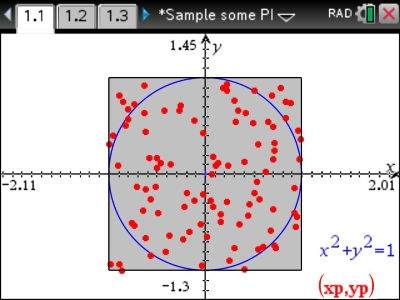# Activities

•• ##### Subject Area

• Standard: VCE: Mathematical Methods: Mathematical Methods

• ##### AuthorAust Senior

45 Minutes

• ##### Device
• TI-Nspire™
• TI-Nspire™ CAS
• TI-Nspire™ Navigator™
• ##### Software

TI-Nspire™
TI-Nspire™ CAS
TI-Nspire™ CAS Navigator™ NC System
TI-Nspire™ Navigator™ NC System

4.4

## Sample Some PI Monte#### Activity Overview

In this activity, simulated random sampling is used to develop the concept of the sample proportion as an estimator of the population proportion. Simulation will allow us to investigate how a sample proportion varies from sample to sample.

#### Objectives

• To appreciate variability in the results obtained from different random samples
• To investigate estimates of a population proportion from sample proportions
• To establish the idea of the sample proportion as a random variable
• Informally investigate the standard deviation of the sampling distribution

#### Vocabulary

population parameter, population proportion, random sample, sample statistic, sample proportion, random variable, estimation